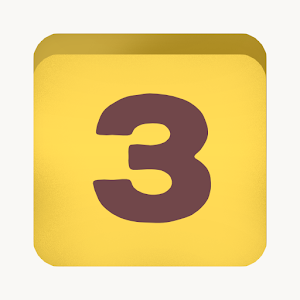\$1.99

# Android Giveaway of the Day - Numerico - Math Cross Game

Solve numbers and mathematical symbols.
\$1.99 期限切れ
ユーザーの評価: 0 (0%) 0 (0%)

このgiveawayオファーはすでに期限が切れています。Numerico - Math Cross Gameが今紹介されています。

Are you good at math? Can you handle some multiplications and subtractions?

In Numerico, the Players are given a board, similar to one found in CrossWords, filled with numbers and mathematical symbols. The player must rearrange the pieces in any way they want in order to make all equations valid.

Mental Lab Games

Puzzle

1.0

19M

Everyone

4.1 and up

## コメント Numerico - Math Cross Game

Thank you for voting!